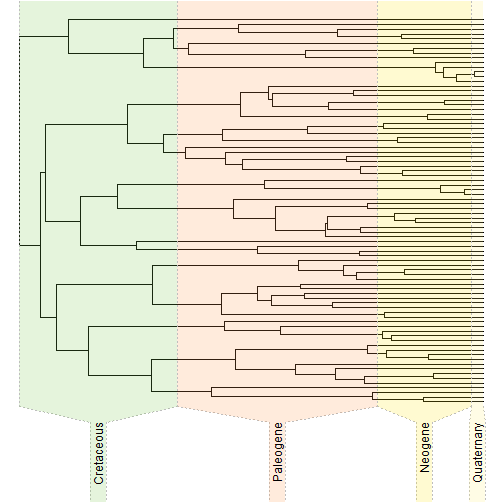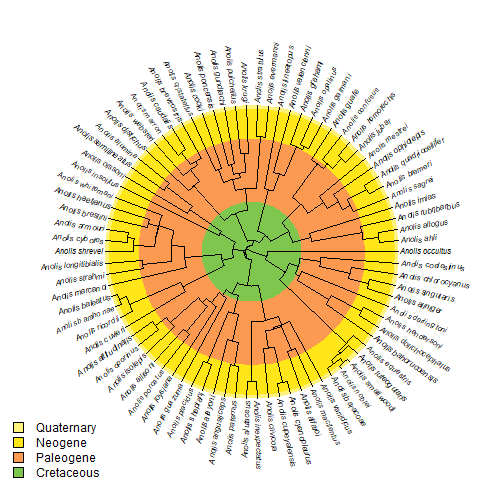## Wednesday, January 3, 2018

### Adding a geological legend to a fan-style tree using concentric circles

“Does anyone know of a function to plot a geologic time scale as a series of concentric circles on a circularly plotted tree?”

Here is a demo using solid, rather than semi-transparent (as in `geo.legend` colors. This avoids the problem of having to plot 'donuts' rather than filled circles. The former scenario I will consider later.

Note that in the following tree - though a genuine empirical phylogeny - has been arbitrarily rescaled to have a total depth of 100 my for illustrative purposes only!

``````library(phytools)
``````
``````## Loading required package: ape
``````
``````## Loading required package: maps
``````
``````library(plotrix)
tree
``````
``````##
## Phylogenetic tree with 82 tips and 81 internal nodes.
##
## Tip labels:
##  Anolis_ahli, Anolis_allogus, Anolis_rubribarbus, Anolis_imias, Anolis_sagrei, Anolis_bremeri, ...
##
## Rooted; includes branch lengths.
``````
``````plotTree(tree,ftype="off",ylim=c(-0.2*Ntip(tree),Ntip(tree)),lwd=1,
xlim=c(max(nodeHeights(tree)),0),direction="leftwards")
obj<-geo.legend() ## this is just to get the colors
````````````r<-max(obj\$leg[,1])-obj\$leg[,2]

plotTree(tree,type="fan",fsize=0.7,lwd=1,ftype="i")
for(i in 1:nrow(obj\$leg)){
color<-paste(strsplit(obj\$colors[i],"")[][1:7],collapse="")
}
par(fg="transparent")
par(fg="black")

function(x) paste(strsplit(x,"")[][1:7],collapse="")),
prompt=FALSE,x=0.95*par()\$usr,y=0.7*par()\$usr)
``````Neat.

#### 1 comment:

1.This comment has been removed by the author.

Note: due to the very large amount of spam, all comments are now automatically submitted for moderation.